# skimage¶

Image Processing for Python

scikit-image (a.k.a. skimage) is a collection of algorithms for image processing and computer vision.

The main package of skimage only provides a few utilities for converting between image data types; for most features, you need to import one of the following subpackages:

## Subpackages¶

color

Color space conversion.

data

Test images and example data.

draw

Drawing primitives (lines, text, etc.) that operate on NumPy arrays.

exposure

Image intensity adjustment, e.g., histogram equalization, etc.

feature

Feature detection and extraction, e.g., texture analysis corners, etc.

filters

Sharpening, edge finding, rank filters, thresholding, etc.

graph

Graph-theoretic operations, e.g., shortest paths.

io

Reading, saving, and displaying images and video.

measure

Measurement of image properties, e.g., similarity and contours.

morphology

Morphological operations, e.g., opening or skeletonization.

restoration

Restoration algorithms, e.g., deconvolution algorithms, denoising, etc.

segmentation

Partitioning an image into multiple regions.

transform

Geometric and other transforms, e.g., rotation or the Radon transform.

util

Generic utilities.

viewer

A simple graphical user interface for visualizing results and exploring parameters.

## Utility Functions¶

img_as_float

Convert an image to floating point format, with values in [0, 1]. Is similar to img_as_float64, but will not convert lower-precision floating point arrays to float64.

img_as_float32

Convert an image to single-precision (32-bit) floating point format, with values in [0, 1].

img_as_float64

Convert an image to double-precision (64-bit) floating point format, with values in [0, 1].

img_as_uint

Convert an image to unsigned integer format, with values in [0, 65535].

img_as_int

Convert an image to signed integer format, with values in [-32768, 32767].

img_as_ubyte

Convert an image to unsigned byte format, with values in [0, 255].

img_as_bool

Convert an image to boolean format, with values either True or False.

dtype_limits

Return intensity limits, i.e. (min, max) tuple, of the image’s dtype.

 skimage.dtype_limits(image[, clip_negative]) Return intensity limits, i.e. skimage.ensure_python_version(min_version) skimage.img_as_bool(image[, force_copy]) Convert an image to boolean format. skimage.img_as_float(image[, force_copy]) Convert an image to floating point format. skimage.img_as_float32(image[, force_copy]) Convert an image to single-precision (32-bit) floating point format. skimage.img_as_float64(image[, force_copy]) Convert an image to double-precision (64-bit) floating point format. skimage.img_as_int(image[, force_copy]) Convert an image to 16-bit signed integer format. skimage.img_as_ubyte(image[, force_copy]) Convert an image to 8-bit unsigned integer format. skimage.img_as_uint(image[, force_copy]) Convert an image to 16-bit unsigned integer format. Do a keyword search on scikit-image docstrings. skimage.data Standard test images. skimage.util

## dtype_limits¶

skimage.dtype_limits(image, clip_negative=False)[source]

Return intensity limits, i.e. (min, max) tuple, of the image’s dtype.

Parameters
imagendarray

Input image.

clip_negativebool, optional

If True, clip the negative range (i.e. return 0 for min intensity) even if the image dtype allows negative values.

Returns
imin, imaxtuple

Lower and upper intensity limits.

## ensure_python_version¶

skimage.ensure_python_version(min_version)[source]

## img_as_bool¶

skimage.img_as_bool(image, force_copy=False)[source]

Convert an image to boolean format.

Parameters
imagendarray

Input image.

force_copybool, optional

Force a copy of the data, irrespective of its current dtype.

Returns
outndarray of bool (bool_)

Output image.

Notes

The upper half of the input dtype’s positive range is True, and the lower half is False. All negative values (if present) are False.

## img_as_float¶

skimage.img_as_float(image, force_copy=False)[source]

Convert an image to floating point format.

This function is similar to img_as_float64, but will not convert lower-precision floating point arrays to float64.

Parameters
imagendarray

Input image.

force_copybool, optional

Force a copy of the data, irrespective of its current dtype.

Returns
outndarray of float

Output image.

Notes

The range of a floating point image is [0.0, 1.0] or [-1.0, 1.0] when converting from unsigned or signed datatypes, respectively. If the input image has a float type, intensity values are not modified and can be outside the ranges [0.0, 1.0] or [-1.0, 1.0].

### Examples using skimage.img_as_float¶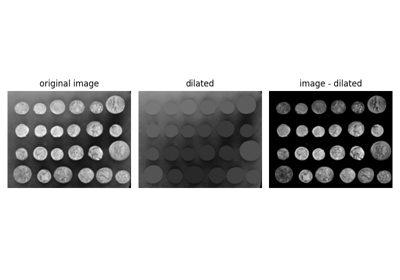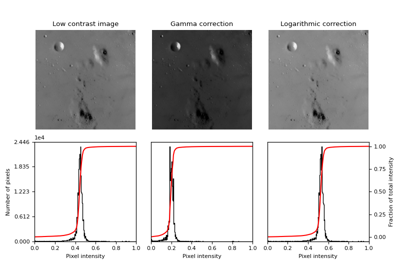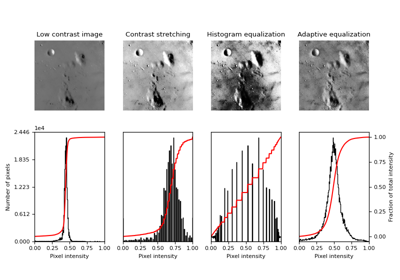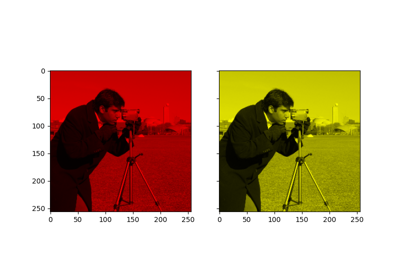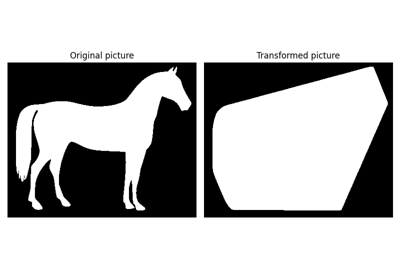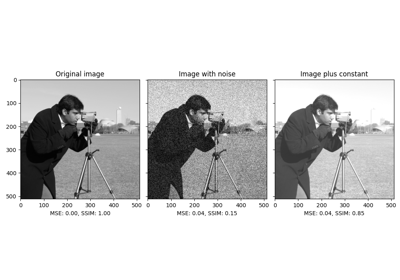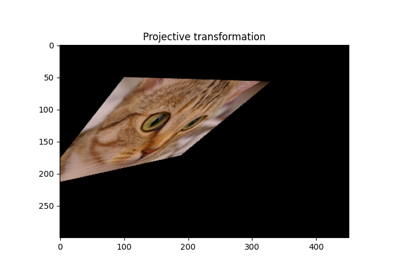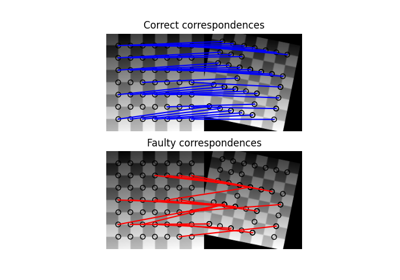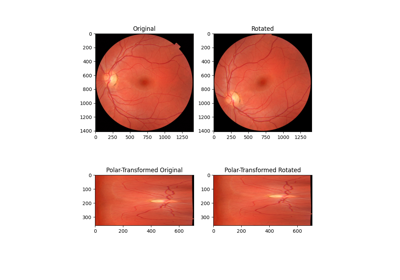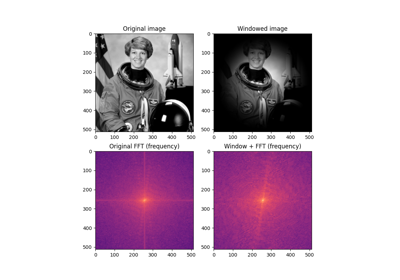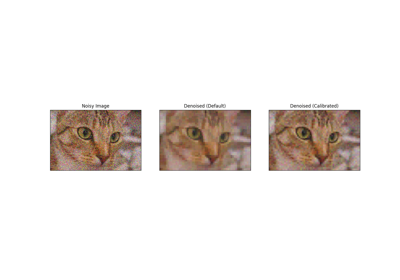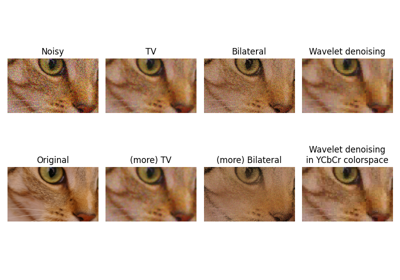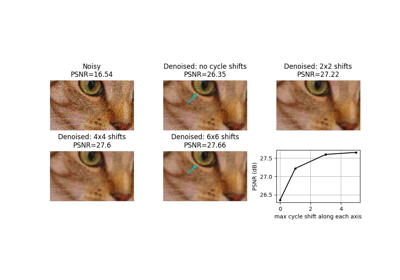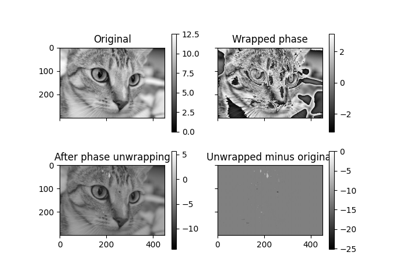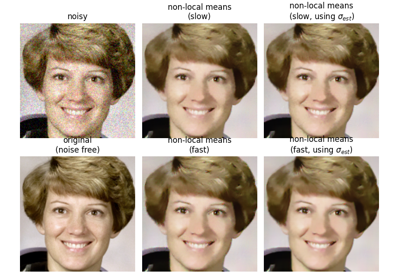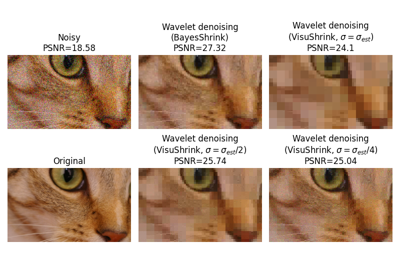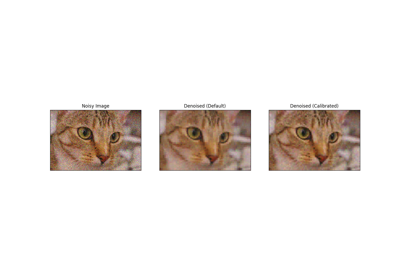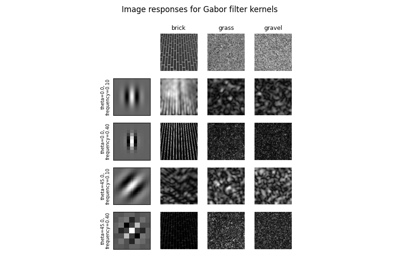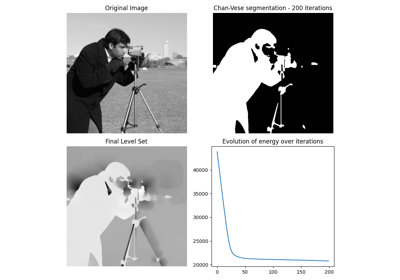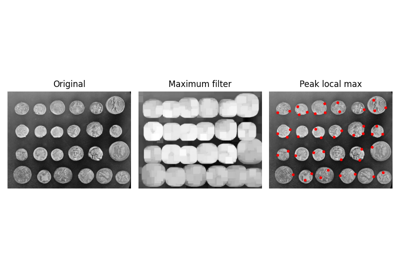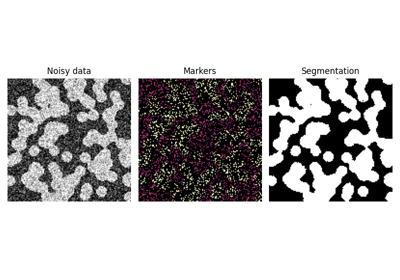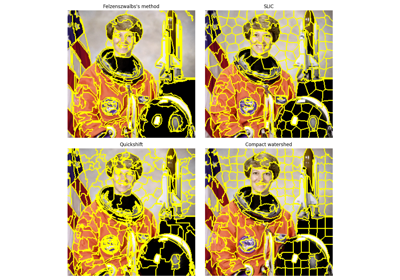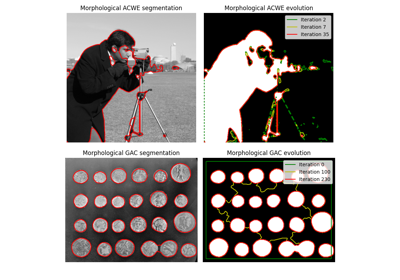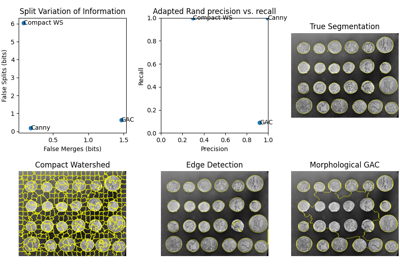## img_as_float32¶

skimage.img_as_float32(image, force_copy=False)[source]

Convert an image to single-precision (32-bit) floating point format.

Parameters
imagendarray

Input image.

force_copybool, optional

Force a copy of the data, irrespective of its current dtype.

Returns
outndarray of float32

Output image.

Notes

The range of a floating point image is [0.0, 1.0] or [-1.0, 1.0] when converting from unsigned or signed datatypes, respectively. If the input image has a float type, intensity values are not modified and can be outside the ranges [0.0, 1.0] or [-1.0, 1.0].

## img_as_float64¶

skimage.img_as_float64(image, force_copy=False)[source]

Convert an image to double-precision (64-bit) floating point format.

Parameters
imagendarray

Input image.

force_copybool, optional

Force a copy of the data, irrespective of its current dtype.

Returns
outndarray of float64

Output image.

Notes

The range of a floating point image is [0.0, 1.0] or [-1.0, 1.0] when converting from unsigned or signed datatypes, respectively. If the input image has a float type, intensity values are not modified and can be outside the ranges [0.0, 1.0] or [-1.0, 1.0].

## img_as_int¶

skimage.img_as_int(image, force_copy=False)[source]

Convert an image to 16-bit signed integer format.

Parameters
imagendarray

Input image.

force_copybool, optional

Force a copy of the data, irrespective of its current dtype.

Returns
outndarray of uint16

Output image.

Notes

The values are scaled between -32768 and 32767. If the input data-type is positive-only (e.g., uint8), then the output image will still only have positive values.

## img_as_ubyte¶

skimage.img_as_ubyte(image, force_copy=False)[source]

Convert an image to 8-bit unsigned integer format.

Parameters
imagendarray

Input image.

force_copybool, optional

Force a copy of the data, irrespective of its current dtype.

Returns
outndarray of ubyte (uint8)

Output image.

Notes

Negative input values will be clipped. Positive values are scaled between 0 and 255.

### Examples using skimage.img_as_ubyte¶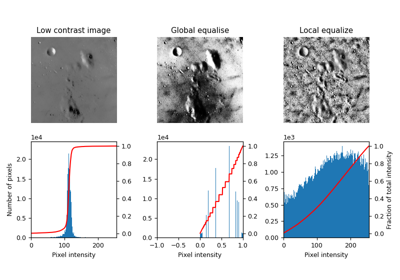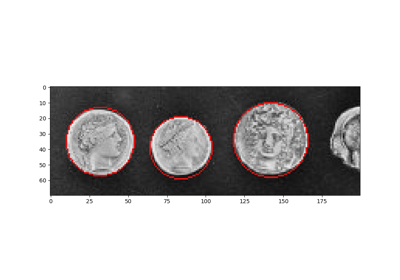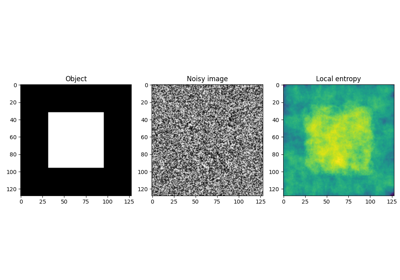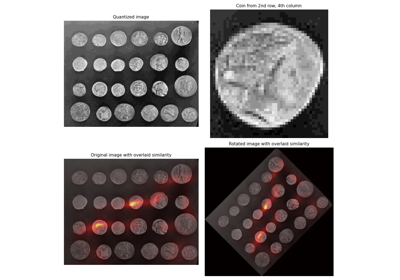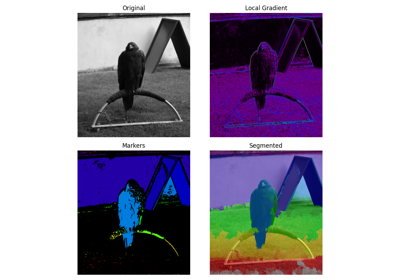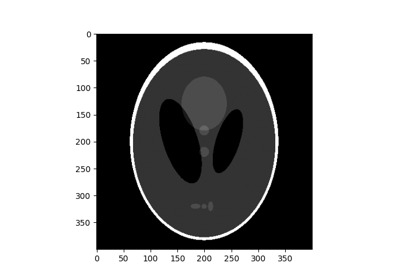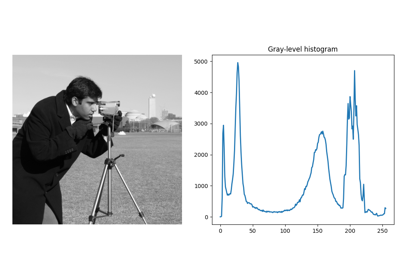## img_as_uint¶

skimage.img_as_uint(image, force_copy=False)[source]

Convert an image to 16-bit unsigned integer format.

Parameters
imagendarray

Input image.

force_copybool, optional

Force a copy of the data, irrespective of its current dtype.

Returns
outndarray of uint16

Output image.

Notes

Negative input values will be clipped. Positive values are scaled between 0 and 65535.

## lookfor¶

skimage.lookfor(what)[source]

Do a keyword search on scikit-image docstrings.

Parameters
whatstr

Words to look for.

Examples

>>> import skimage
>>> skimage.lookfor('regular_grid')
Search results for 'regular_grid'
---------------------------------
skimage.lookfor
Do a keyword search on scikit-image docstrings.
skimage.util.regular_grid
Find n_points regularly spaced along ar_shape.